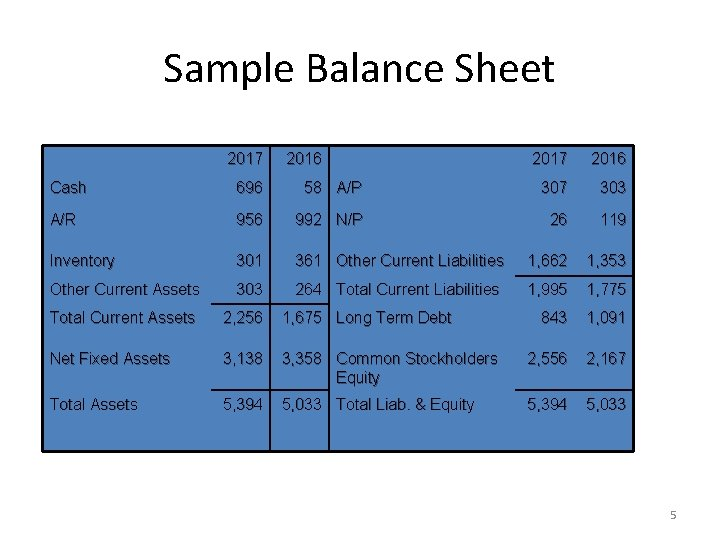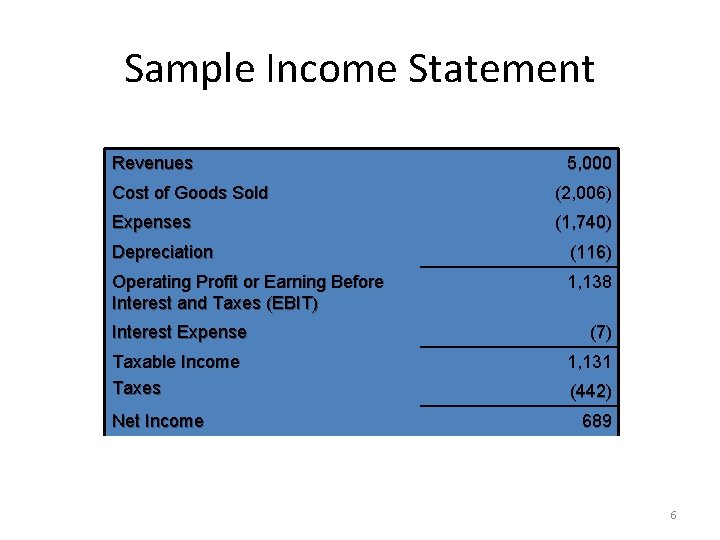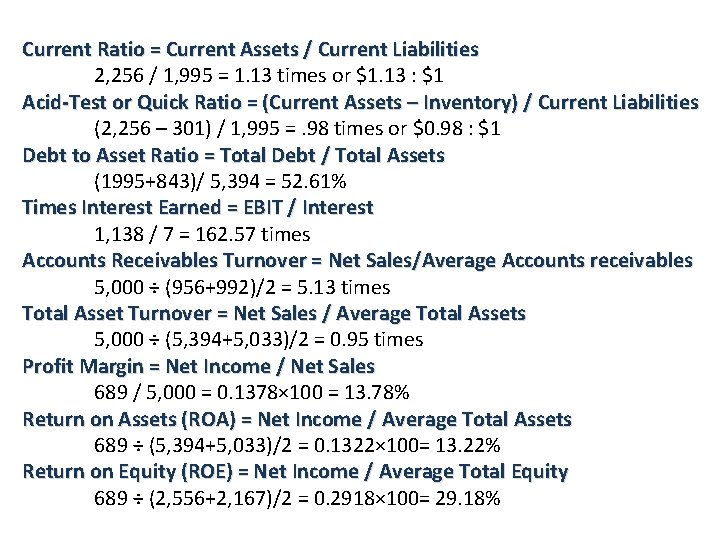# Ratio Analysis Chapter 18 Ratio Analysis Ratio analysis

• Slides: 7Ratio Analysis Chapter 18Ratio Analysis Ratio analysis involves methods of calculating and interpreting financial ratios to analyze and monitor the firm’s performance. Ø Ratios allow for better comparison through time (Time series or time trend analysis) or between companies (Peer group or cross sectional analysis) Ø As we look at each ratio, ask yourself what the ratio is trying to measure and why that information is important Ø Ratios are used both internally and externally 2Using Financial Ratios: Interested Parties • Current and prospective shareholders are interested in the firm’s current and future level of risk and return, which directly affect share price. • Creditors are interested in the short-term liquidity of the company and its ability to make interest and principal payments. • Management is concerned with all aspects of the firm’s financial situation, and it attempts to produce financial ratios that will be considered favorable by both owners and creditors. 3 -3Using Financial Ratios: Types of Ratio Comparisons • Cross-sectional analysis is the comparison of different firms’ financial ratios at the same point in time; involves comparing the firm’s ratios to those of other firms in its industry or to industry averages • Time-series analysis is the evaluation of the firm’s financial performance over time using financial ratio analysis • Comparison of current to past performance, using ratios, enables analysts to assess the firm’s progress. • Developing trends can be seen by using multiyear comparisons. • The most informative approach to ratio analysis combines cross-sectional and time-series analyses. 3 -4Sample Balance Sheet Numbers in millions 2017 2016 Cash 696 58 A/P 307 303 A/R 956 992 N/P 26 119 Inventory 301 361 Other Current Liabilities 1, 662 1, 353 Other Current Assets 303 264 Total Current Liabilities 1, 995 1, 775 Total Current Assets 2, 256 1, 675 Long Term Debt 843 1, 091 Net Fixed Assets 3, 138 3, 358 Common Stockholders Equity 2, 556 2, 167 Total Assets 5, 394 5, 033 Total Liab. & Equity 5, 394 5, 033 5Sample Income Statement Numbers in millions, except EPS & DPS Revenues 5, 000 Cost of Goods Sold (2, 006) Expenses (1, 740) Depreciation (116) Operating Profit or Earning Before Interest and Taxes (EBIT) 1, 138 Interest Expense Taxable Income Taxes Net Income (7) 1, 131 (442) 689 6Current Ratio = Current Assets / Current Liabilities 2, 256 / 1, 995 = 1. 13 times or \$1. 13 : \$1 Acid-Test or Quick Ratio = (Current Assets – Inventory) / Current Liabilities (2, 256 – 301) / 1, 995 =. 98 times or \$0. 98 : \$1 Debt to Asset Ratio = Total Debt / Total Assets (1995+843)/ 5, 394 = 52. 61% Times Interest Earned = EBIT / Interest 1, 138 / 7 = 162. 57 times Accounts Receivables Turnover = Net Sales/Average Accounts receivables 5, 000 ÷ (956+992)/2 = 5. 13 times Total Asset Turnover = Net Sales / Average Total Assets 5, 000 ÷ (5, 394+5, 033)/2 = 0. 95 times Profit Margin = Net Income / Net Sales 689 / 5, 000 = 0. 1378× 100 = 13. 78% Return on Assets (ROA) = Net Income / Average Total Assets 689 ÷ (5, 394+5, 033)/2 = 0. 1322× 100= 13. 22% Return on Equity (ROE) = Net Income / Average Total Equity 689 ÷ (2, 556+2, 167)/2 = 0. 2918× 100= 29. 18%